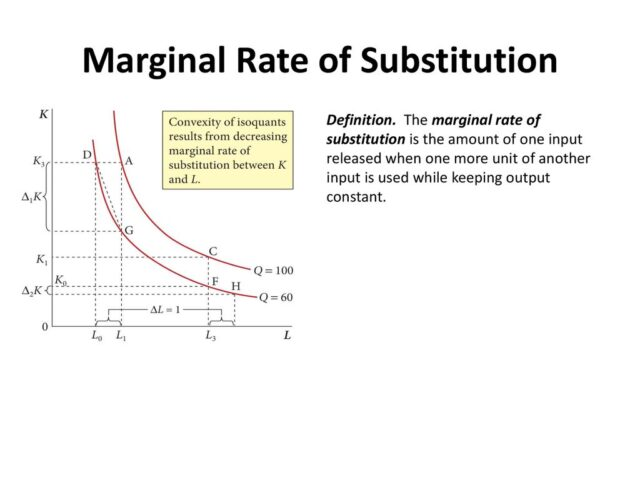# Marginal Rate of Substitution

## Marginal rate of Substitution

In general language, the Marginal Rate of Substitution also known as MRS is simply the number of goods a consumer will take before choosing the other competition of this product. It stands for the actual amount which the consumer will like to buy or consume in comparison with the other listed product. It shows up to what quantity the consumer will prefer a certain product over its competition if the other product is equally satisfying in terms of value and performance.

The major use of obtaining a marginal substitution rate is to establish and observe a stable relationship between two competitive goods without compromising any kind of satisfying factor of the consumer. The value of MRS helps in determining whether the substitution between these products will cause positive, negative or no impact on consumer behaviour and satisfaction.

While studying the indifference theory of goods, MRS is used to examine the consumer behaviour about different goods to achieve the same level of self-satisfaction. It helps the analysing team to obtain a clear picture of how a product can be substituted with another one without troubling the consumer Services.

Whereas if we start plotting MRS value on a graph sheet, we will have to draw indifference curves for both the products in consideration. By plotting these two indifference curves in the same sheet, we can acquire a clearer picture of how the MRS value is useful.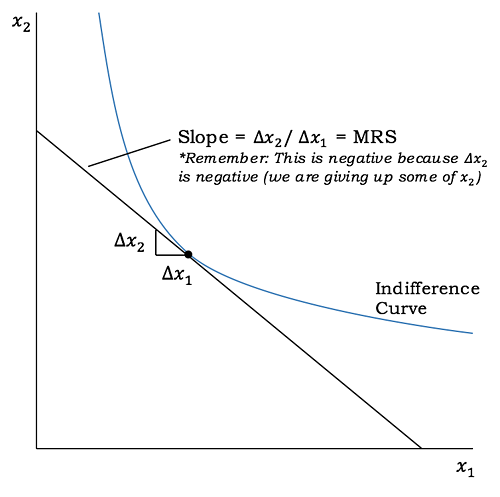## How To Work With MRS

The marginal rate of Substitution is one of the basic and initial values you may need to study consumer behaviour on different products. There are many situations in which a consumer might want to switch from one product to another. It can be either lack of availability or a sudden hike in the prices of the product. These factors can lead to the substitution of one product with another. But by how much quantity, in which situation and how to manage it, are some questions solved by calculating and obtaining an accurate MRS value. As MRS value will help in determining the trends in consumer behaviour and interest of the consumer in a certain product.

In more common words, MRS indicates the number of units in which you can substitute a certain product with another to achieve the same results. This can be due to the lack of Scarcity of a certain product where you have no option other than substituting the product with its complementary product to achieve the same goal.

Whereas when you plot the indifference curve, you will obtain values in which you can combine both entities for the final result to be equally satisfying. However, you may obtain different values of MRS by simply putting the values in its basic formula. You can use this method to observe consumer behaviour without any heavy mathematical calculations.

Read a full Guide on Rule of 70 which helps you to make your investment and trading better.## How the MRS value can be examined

The most common way of studying and understanding MRS value is via Graph. The economists form a graph as an indifference curve for each of the considered products. Then, the actual slope of these two entities is examined to obtain the value of MRS. These slopes give you the nature, type and trend of MRS value between the two considered products. Simply, the MRS value is equivalent to the slope obtained by indifference curves of these products.

This slope provides you with information about the trend of MRS value to examine if it’s stable. If not, then in which direction the trend of mrs value is moving. According to the highest order indifference curves of two products, it can move in three basic ways.

• The first way for an indifference curve trend to move is to go downward. Also called a diminishing graph or diminishing indifference curve. It shows that the substitution is not useful for the consumer and will not yield expected results. It is shown as bending towards the origin on the graph sheet In a convex manner.
• The second type of deflection seen in indifference curves is a straight line. It indicates perfect substitution. It shows how a product can be perfectly substituted by the other product under study without Compromising with the satisfying criterion of the consumer. It can be useful to give the perfect substitution options to the consumers.
• The last type or way in which the indifference curve can bend is increasing way. The graph obtained under this criterion is in L shape with a perfect 90 degrees. It shows how the other product works better than the substituted one. It shows how the products are Complementary instead of competition or substitution to each other.

This way, you can obtain a clear analysis and an idea about the consumer behaviour for different products by simply drawing their indifference curves. A product must be above the satisfying line to be called a good substitution to the other product.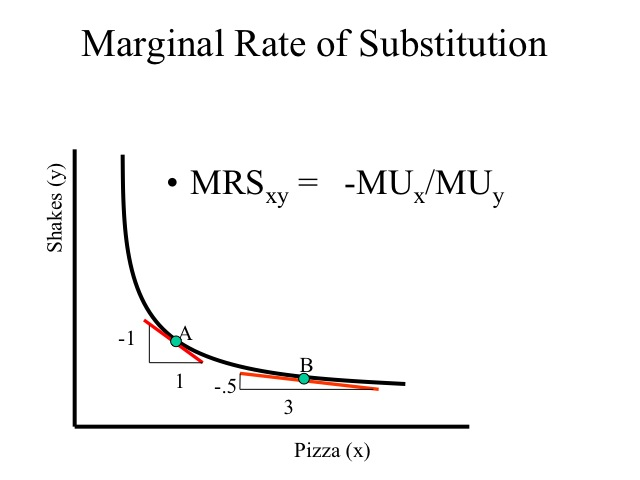## What are the Factors for Diminishing Marginal Rate of Substitution

By Diminishing the Rate of substitution, it’s meant that for the decrease in the availability of one entity, the demand of the other one increases. If the second product is able to cover the difference created by the unavailability of the first one, only then the Diminishing Rule of MRS is applicable.

However, there are certain assumptions made by the economists to represent the diminishing Marginal Rate of substitution for two products. The major assumptions made for representing diminishing MRS are:

• None of the axis present on the graph are intersected by the indifference curve at any point or for any possible value
• There is no point of intersection between two different indifference curves belonging to different product performances.
• The indifference curve represented on the graph bends towards the origin i.e. it should be downward moving.
• The slope of the indifference curve must be negative or downwards to indicate that the MRS value is diminishing.
• The other products or substitutes never fit perfectly in the picture. They are neither perfect substitutes nor a complimentary substitute for the product under consideration
• The change in quantity for each entity will be made in accordance with each other. If one goes up, the other entity goes down. And vice versa. The quantity of both the products will not be increased together.
• After making these assumptions, you can simply apply the basic formula of calculating MRS for two products in the market.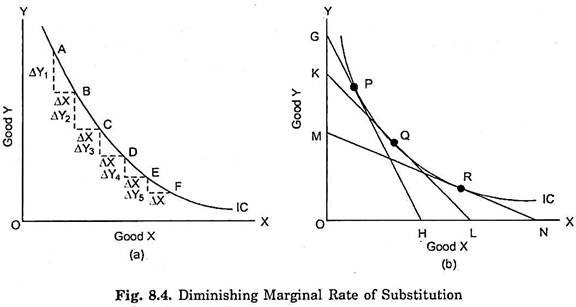## How does MRS relate to the Indifference curve?

In the simplest possible way, MRS can be defined as the slope of the given indifference curve at a certain point along the curve. It is the change in values i.e. the demand of any product with comparison to others, obtained by taking the slope of the curve at a certain point. The curvature of the indifference curve is a major factor in deciding the value of MRS. It also indicates the nature, type, and kind of MRS for the given two products.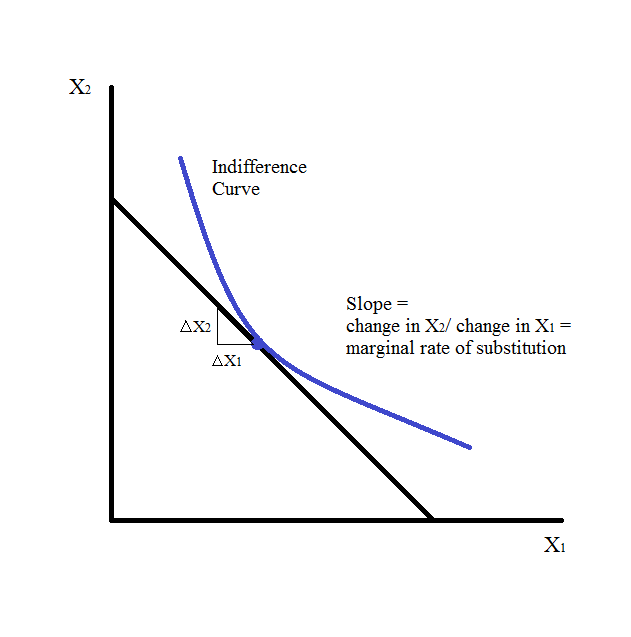## How to Calculate MRS

Calculating MRS does not require high-level mathematics or knowledge of accounts. You can simply use the basic and universal formula of MRS for calculating accurate MRS values for two products.

Say, we have two products in consideration named A and B, the formula applied to calculate MRS(A, B) Is simply given by the change in demand of A divided by Change in demand of B.

Where B is the product already in existence and play a role of getting substituted by other product in this case. However, A is the second product or simply the substitute for B.

The basic formula of MRS provides you with the idea that the change in demand for one product is indirectly proportional to the demand for another product. This means if the demand of one increases, the demand for the other will decrease and vice versa.

You can also obtain an underlying statement about which way the indifference curve will bend if the value of MRS is more than one or less than one. For every higher value of MRS, it is understood that the demand for one product is rightfully satisfied by the availability of another.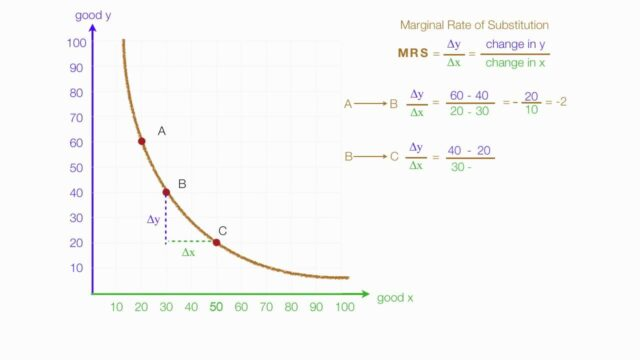## Limitations of MRS

MRS simply compares two products regarding their compatibility to fulfil respective demands in the market and maintain balance. However, the biggest limitation is that you can only compare two products at a time while using MRS value. If you wish to compare more than two products at the same time, it becomes difficult and confusing. The only way to resolve this issue is by finding out the relations between these products. This becomes lengthy and troublesome for economists.

Another major limitation of MRS is that it assumes that the utility and performance of both the products are the same which is not possible in real-life scenarios. The two different products have different impacts and valuations in the eyes of consumers which can be almost the same but never identical.

## Conclusion

The marginal rate of substitution is one of the basic factors you need to keep in check while analysing consumer behaviour. It shows how a consumer reacts and changes their choices while one product is unavailable to keep the satisfaction level the same. It’s an easy, useful and accurate method of determining consumer response to changing markets.

• How can I calculate the Marginal Rate of Substitution?

You can easily calculate the marginal Substitution with the formula:

MRSxy= change in Y/change in X. Here, it simply implies the indifference curve between the two.

•  What is MRT in Economics?

MTS stands for Marginal Rate of transformation. It helps the economists to check and verify the cost for producing one more unit of the product. In such a scenario, the opportunity cost can be represented according to the lost production of other goods.

• Explain the difference between the marginal rate of transformation and the marginal rate of substitution?

When you talk about the marginal rate of substitution, it analyses how willing the customer is to replace one product with another. But, when it comes to the marginal rate of transformation, it analyses the amount of willingness of the manufacturer to reduce the production cost for other products.

• What is the difference between MRS and MTRS?

So, the basic difference between the MRS and MTRS is that the MTRS is more about focusing on the producer equilibrium and MRS is all about the consumer equilibrium. When talking about MTRS, you need to maintain a give and take relationship to keep things constant.